## Example Questions

### Example Question #1 : How To Find The Surface Area Of A Cube

A rectangular box is 6 feet wide, 3 feet long, and 2 feet high.  What is the surface area of this box?

48 sq ft

36 sq ft

22 sq ft

72 sq ft

64 sq ft

72 sq ft

Explanation:

The surface area formula we need to solve this is 2ab + 2bc + 2ac.  So if we let a = 6, b = 3, and c = 2, then surface area:

= 2(6)(3) + 2(3)(2) +2(6)(2)

= 72 sq ft.

### Example Question #1 : How To Find The Surface Area Of A Cube

What is the surface area of a rectangular box that is 3 feet high, 6 feet long, and 4 feet wide?

72

96

108

144

84

108

Explanation:

Surface area of a rectangular solid

= 2lw + 2lh + 2wh

= 2(6)(4) + 2(6)(3) + 2(4)(3)

= 108

### Example Question #1 : How To Find The Surface Area Of A Cube

A large cube is made by fitting 8 smaller, identical cubes together. If the volume of each of the smaller cubes is 27, what is the surface area of the large cube?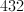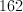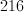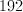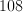Explanation:

Since the volume of the smaller cubes with edges,, is 27, we have: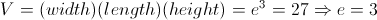.

The large cube has edges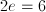.

So the surface area of the large cube is: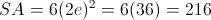.

### Example Question #11 : Cubes

Quantity A:

The surface area of a cube with a volume of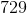.

Quantity B:

The volume of a cube with a surface area of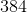.

The relationshp cannot be determined from the information given.

Quantity B is greater.

Both quantities are equal.

Quantity A is greater.

Quantity B is greater.

Explanation:

The relationship can be determined, because it is possible to find the surface area of a cube from the volume and vice versa.

Quantity A:

To find the surface area of the cube, you must find the side length. To find the side length from the volume, you must find the cube root.

Find the cube root of the volume.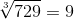Insert into surface area equation.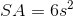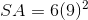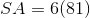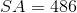Quantity B:

To find the volume of a cube, you must find the side length. To find the side length from the surface area, you must divide by 6, then find the square root of the result. Then, cube that result.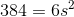Divide by 6.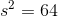Square root.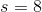Now, to find the volume.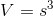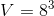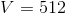Quantity B is greater.

### All GRE Math Resources